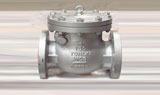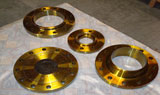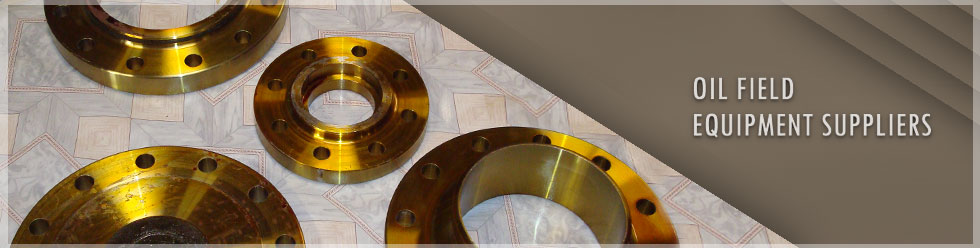### equation and conversion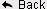Formula to calculate (Appr. Weight) Kg per meter (Kg/m) for steel round pipe and tubing

M= (D-T) x T x 0.02466
Where M = mass to the nearest 0.01 Kg/m
D= Outside Diameter in millimeters (OD in mm)
(To nearest 0.1 mm for OD up to 406.4 mm)
(To nearest 1.0 mm for OD 457 mm and above)
T = Wall thickness to nearest .01 mm

Volume Of a cylinder

A cylinder with radius r units and height h units has a volume of V cubic units given by
Volume V = Pie r2 h where Pie = 3.14, r = radius and h = height

Conversions

Foot to Meter
1 Foot = 0.348 Meters

Meter to Feet
1 Meter = 3.28 Feet

Foot to Inches
1 Foot = 12 Inches

Inches to Foot
I Inch = 0.833333 Feet

Mille meter to Inches
1MM = 0.03937 Inches

Inch to mille meters
1 Inch = 25.4 MM

Pound to kilograms
1 Pound = 0.453593237 Kg

Kilogram to Pounds
1 Kg = 2.2046 Pounds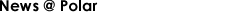• Iraq considering BP proposal for Kirkuk oilfield ..
• ADIPEC 2013 From Nov 10-13 ..

### PRODUCTS